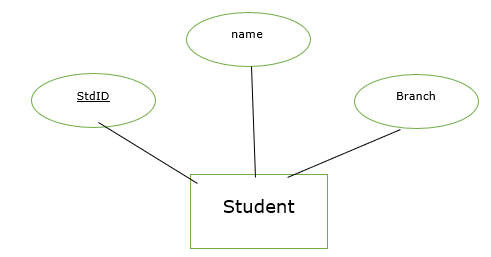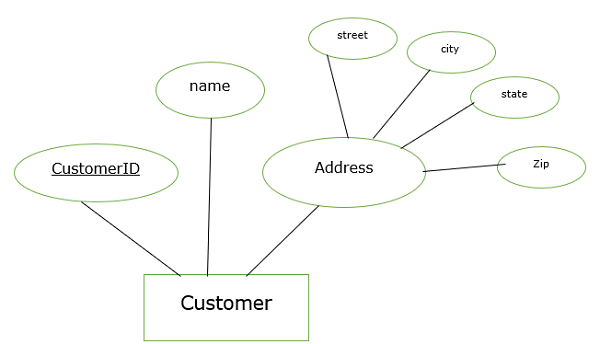# What is a composite attribute in DBMS?

The attributes that cannot be divided into sub-parts are called simple attributes. The attributes which can be divided into sub-parts are called composite attributes.

## Example

• Balance, account number are simple attributes

• Name, address is called composite attributes

Let’s consider an example of how to convert Entity Relationship (ER) model of composite attribute to Relational model.

## Methods for mapping

Name of relation = Entity set of ER diagram

Name of column = Attribute of ER diagram

## Example

In this example,

• Name of the relation =student

• Name of columns=StdID, name, BranchThe equivalent relation for the above ER diagram is −

### Student

 StdID Name Branch

Now apply this technique for Mapping ER diagram with a composite attribute as shown below −In the above example composite attribute is address and component attributes are street, city, state, zip.

The equivalent relation for the above ER diagram is −

### Customer

 CustomerID Name Street City State zip